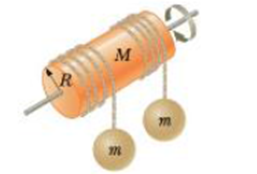Chapter 8, Problem 87AP

Chapter
Section
Textbook Problem

A uniform solid cylinder of mass M and radius R rotates on a friction-less horizontal axle (Fig. P8.87). Two objects with equal masses m hang from light cords wrapped around the cylinder. If the system is released from rest, find (a) the tension in each cord and (b) the acceleration of each object after the objects have descended a distance h.Figure P8.87

(a)

To determine
The magnitude of tension in each cord.

Explanation

Given Info:

Mass of the cylinder is M, mass of the objects is m; radius of the cylinder is R.

Explanation:

Consider an axis passes through the centre of the cylinder. Weight of both the masses exerts torque on the cylinder.

Formula to calculate torque on the cylinder is,

τ=2TR (1)

• τ is the torque acting on the cylinder
• T is the tension on the two cords
• R is the radius of the cylinder

Formula two calculate torque on the cylinder in terms of angular acceleration is given by

τ=Iα (2)

• I is the moment of inertia of the cylinder about an axis passes through the centre of the cylinder
• α is the angular acceleration

Formula to calculate the moment of inertia of the cylinder is given by

I=12MR2

Substitute the above expression for I in (2) to calculate torque

τ=12MR2α (3)

Formula for angular acceleration is,

a=αR

• a is the downward acceleration of the masses

Rewrite the equation (3) in terms of a

τ=12MR2(aR)=12MRa (4)

Equate (1) and (4) to calculate T

2TR=12MRaT=14Ma

Let us consider downward as positive

(b)

To determine
The magnitude of acceleration on each object.

Still sussing out bartleby?

Check out a sample textbook solution.

See a sample solution

The Solution to Your Study Problems

Bartleby provides explanations to thousands of textbook problems written by our experts, many with advanced degrees!

Get Started

Adolescents are likely to grow out of early disordered eating behaviors by young adulthood. T F

Nutrition: Concepts and Controversies - Standalone book (MindTap Course List)

What are the moral implications of using ergogenic aids?

Understanding Nutrition (MindTap Course List)

How may our senses be enhanced?

An Introduction to Physical Science